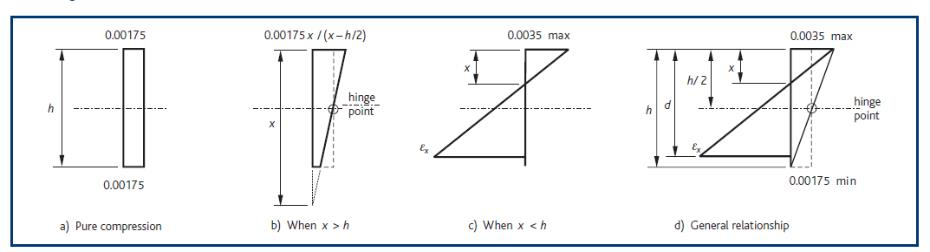# Bending and axial force

In determining the resistance of sections in bending with axial force, in addition to the assumptions made for flexure above, the following assumptions are made:

• For sections in pure axial compression, the compressive strain in concrete should be limited to 0.00175, see Figure 9) below.
• For sections in pure flexure, the compressive strain in concrete should be limited to 0.0035, see Figure 7).
• For situations intermediate between these two conditions, the strain profile is defined by assuming that the strain is 0.00175 at half the depth of the section, see Figure 9b).

#### Figure 9: Strain diagrams for columns (valid for concrete up to class C50/60 from Eurocode 2)Extract from How to Design Concrete Structures using Eurocode 2: Columns (page 44, Figure 9)

The reinforcement for columns may be determined in two ways:

• Iteration on neutral axis depth, x (see Figure 8) such that Asreqd for bending = Asreqd for axial load.
• Column charts based on NEd/bhfck vs MEd/bh2fck and d2/h.

#### Figure 8: Stress block diagram for columnsExtract from How to Design Concrete Structures using Eurocode2: Columns (page 43, Figure 8)

Axial forces are determined from analysis. Design moments must include for 1st order moments from analysis and for the effects of imperfections and, in the case of slender columns, for the effects of slenderness.

For more explanation see information on